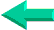### This is an excerpt from Pierre Leclerc (www.excel-vba.com)

The 2 Most Important

Excel Functions AND
Formulas

SUMPRODUCT

AND

INDEX/MATCH

# Lesson 9: Excel Spreadsheets Statistical Functions (83)

In annex 9 you have found a description of all 83 Excel functions in the "Statistical" category. Below is the list of the 7 most useful ones.

 Functions What it Does AVERAGE Returns the average of its arguments COUNT Counts how many numbers are in the list of arguments AVERAGEA Returns the average of its arguments, including numbers, text, and logical values COUNTA Counts how many values are in the list of arguments) RANK Returns the rank of a number in a list of numbers LARGE Returns the k-th largest value in a data set SMALL Returns the k-th smallest value in a data set New Functions in Excel 2007 AVERAGEIF Calculates the average within a range that meet a given criteria (SUMPRODUCT does better) AVERAGEIFS Calculates the average within a range that meet one or many given criteria (SUMPRODUCT does better) COUNTIFS Counts the number of nonblank cells within a range that meet the given criteria (SUMPRODUCT does better)

LARGE, SMALL

And what if you want the second or third largest value or the second smallest value. Use LARGE and SMALL like this:
=LARGE(A1:A5,2), =LARGE(A1:A5,3), =SMALL(A1:A5,2)
You can use these functions with dates.

As a matter of facts you can forget about MIN and MAX with:
=LARGE(A1:A5,1), =SMALL(A1:A5,1)

COUNT and  COUNTA

If you want to count the number of cells that are not blank COUNT and COUNTA will return a different result if in one of the cells there is a text. OR A SPACE
=COUNT(A1:A5) will return 5 is only numbers OR DATES are present in cells A1 to A5 and 4 if there is a letter, an empty cell OR A SPACE in one of the cells. The SPACE thing is important to remember when you are importing data from an external source.
=COUNTA(A1:A5) will return 5 unless one of the cells is empty. If all the cells contain numbers, letters OR SPACES the result will be 5.

AVERAGE and AVERAGEA

Watch for dates! If you want the average of a range and there is a date within there is a problem because dates are numbers. If all the cells are dates, indeed you can calculate the average date of.... The difference between AVERAGE and AVERAGEA becomes evident when one of the cells contains a text OR A SPACE and don't forget the SPACE. A cell containing a space is NOT empty.Back home